﻿ Robust Cascade Temperature Control for a HSNWTPublications are Open
Access in this journal
Article Versions
Export Article
• Normal Style
• MLA Style
• APA Style
• Chicago Style
Research Article
Open Access Peer-reviewed

### Robust Cascade Temperature Control for a HSNWT

Yongbo Lai , Dewen Kong, Zhiwei Wang
American Journal of Electrical and Electronic Engineering. 2018, 6(3), 93-99. DOI: 10.12691/ajeee-6-3-4
Received September 20, 2018; Revised November 01, 2018; Accepted December 12, 2018

### Abstract

Applies programmable logic controller (PLC) to configure control hardware system, addresses the temperature control for a high speed nitrogen gas wind tunnel (HSNWT) testing, in order to obtain variable high temperature control with variable high speed gas flow, a coordinating factor of the gas flow is proposed between the tunnel’s heating and water cooling systems, moreover, considering the temperature modeling nonlinearities, uncertainties and disturbances, establish robust cascade fuzzy PID and expert predictive control strategies for the inner-loop water cooling subsystem and the outer-loop heating system, respectively, which effectively overcomes the influences of large inertia and transport time delay on the temperature responses. Furthermore, designs human-machine integrated user control interface (HMI), achieves fast and accurately control for user operating. The designed system are simulated and tested in the application, which results demonstrate that the system runs stable and reliable, has strongly robustness when the temperature changes with different loading heat modes, and has excellent capability of variable high speed nitrogen gas flow.

### 1. Introduction

The high speed nitrogen gas wind tunnel (HSNWT) is an important experimental equipment for researching aerodynamics and materials physical properties, widely used in the field of aerospace crafts and advanced instruments researching. The physical properties of experimental materials can be obtained by the wind tunnel testing, reduce the manufacturing cost and risk. For the experimental and applying requirements, in this paper, the temperature control system is designed for a kind of circulating HSNWT, the system is composed of the water cooling system and nitrogen gas heater power control system, which components mainly have two nitrogen gas heaters, one circulating pump, one vacuum pump, one spraying pump, four fans of cooling tower, cooling water pipeline, proportional valves, water flow rate and temperature instruments, sensors and etc., its overall simplified building is shown in Figure 1.

The HSNWT that needs produce a uniform variable and controllable temperature, which the HSNWT temperature control endures the high speed nitrogen gas flow changes, cooling water flow rate and environmental temperature disturbances (and the nitrogen gas wind speed generated which is not considered in this paper).

It must be pointed out that the different high speed nitrogen gas flow are needed at the same temperature testing, the high speed nitrogen gas flow plays an important role when conducting test, it is difficult to measure and control the corresponding temperatures in the HSNWT system with high speed nitrogen gas flow, modeling uncertainties, errors and disturbances. There is a need to develop some mathematical models that takes into accounts the combined dynamics of the aforementioned influences, and control methods for high performance variable temperature control process.

• Figure 1. The simplified scheme of the HSNWT system

For wind tunnel testing control, there were many results in the past few decades, the low speed wind and high temperature wind tunnels were researched in 1, 2, respectively. In 3 a genetic algorithm PID was applied for a high-temperature multiphase flow wind tunnel, Yunhua Li and Chaozhi Cai etc. 4 applied robust cascade fuzzy PID technology achieved a high speed heat-airflow wind tunnel control. Recently, scholars and engineers have presented some novel control techniques for thermal processing in the industry application 5, 6, 7, 8, 9, 10, 11, 12. For complex temperature control systems, modeling and control strategies were researched in 5, 6. In 7 based on BP and GA the static temperature compensation and temperature drift could be reduced properly. Zhang et al. 8 used a novel state space model predictive method to solve the industrial furnace temperature control, reference 10 applied the immune genetic algorithm with BP network resolved the main steam temperature control. For dynamic temperature compensation, Qiao and Chai 11 proposed an intelligence-based temperature switching control implemented in the raw meal production. To eliminate the coupling effect, an intelligent PID decoupleing control system integrating self-growing radial basis function neural network was proposed in 12. Compared with the aforementioned results, PLC-based hardware configuring, this paper studies the variable temperature control for an HSNWT, it synthesizes their advancements in the static temperature drift and dynamic disturbances attenuation, meanwhile, adequately considered the system modeling uncertainties, errors and unknown disturbances.

The sequence of this paper which is organized as follows:

1) A main control circuit is designed for the HSNWT temperature system in section 2.

2) Section 3 which gives out the mathematical models that characterize the dynamics of HSNWT, which offers the essential conditions for numerical simulation and design testing analysis.

3) Fuzzy-PID control laws (consisting a water flow rate and water temperature control inner bi-loops) and expert predictive control strategy (outer-loop nitrogen gas heating) are proposed for the HSNWT variable temperature control process, respectively. Furthermore, for user conveniently operating, the HMI is designed in section 4.

4) Lastly, the designed control system are simulated and tested in the application, demonstrates its robustness and excellent adaptability for variable high-speed gas flow and variable high temperature.

In the sequel, we provide definition of the HSNWT related physical variables in Table 1.

### 2. System Control Hardware Configuring

In Figure 1 the circulating pump and cooling fans power are regulated by variable-frequency drive converters (M430), two high power MOS-controlled transistors (MCT) modules control the nitrogen gas heaters power, spraying pump and vacuum pump work with fixed voltage. The sensors and actuators are physically connected to the PLC, the role of the PLC is to read the sensor signals and convert them into sensor variables that is readable by the control strategy running on the control of industrial personal computer (IPC). Then, in term of set-point values, the PLC converts decision variables from the control IPC into signals and send them to the physical actuators. The system control hardware applies the Siemens PLC with input / output (DI/DO) modules and industrial computer and the HMI to configure, in which the HMI is applied for user operating in the testing, the construction of system control hardware is shown in Figure 2.

• Figure 2. System control hardware configuring

### 3. System Mathematical Modeling

The relatively complete modeling of the HSNWT as a thermal-dynamic system is still a challenge and under exploited for effective temperature control.

3.1. Modeling of Water Flow Rate

The water flow rate is mainly controlled by variable voltage variable frequency M430 in Figure 1, the input control signal of the M430 is the PLC controller output voltage up, the circulating pump’s motor working voltage is M430 output voltage Up. The relationship between control input and output voltages of M430 can be approximately expressed by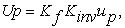(1)

where Kf is the gain between frequency and the input control voltage up, and Kinv is the voltage frequency ratio. According the water flow dynamic and neglecting the influence of the compressibility, the pump output water flow rate qw (m3/s) can be approximately expressed by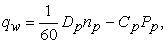(2)

where Pp is the output water pressure of the pump, np is the actual rotational speed per minute. Considering the mechanical and physical specifications of the pump motor and the long cooling water pipeline, neglecting the expansion and compressibility of water, similarly the reference 4 modeling method, the water flow rate qw approximately transfer functions is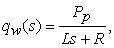(3)

where the water fluid resistance R, capacitance C, and inductance L, Pp is the output pressure of the pump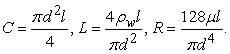From (1) to (3) yields the transfer function Gp(s) between the water flow rate and pump input control voltage up(s) as follows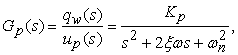(4)

where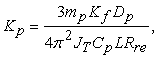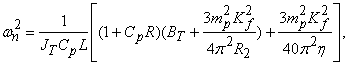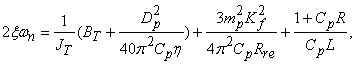and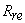,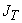,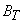,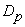,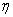see Table 1.

3.2. Modeling of Water Flow Rate

Using the aforementioned water flow rate parameter modeling approach, the transfer function between the cooling fans working voltage Uf (s) and fans rotational speed nf (s) can be expressed by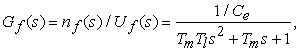(5)

it leads to the transfer function between the cooling water dissipated thermal energy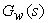and cooling fans control signal up(s) as follows: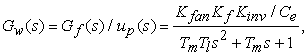(6)

where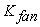is the dissipating coefficient.

3.3. Modeling of Nitrogen Gas Heater

The nitrogen gas heater output heat energy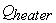and the MCT module output working voltage UMCT approximately transfer functions is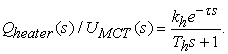(7)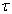is the heat energy transferring delay. The transfer function between MCT modules control signal uh(s) and the output voltage UMCT (s) to heaters can be written as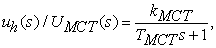(8)

so it leads the transfer function between MCT modules control signal and heat energy as follows: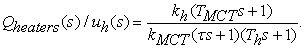(9)
3.4. Modeling of Testing Tunnel Temperature

It is assumed that the nitrogen gas temperature is uniform, by the law of energy conservation, the temperature dynamic model is given in (10)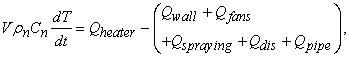(10)

where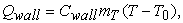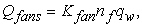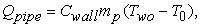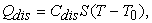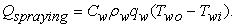Assume that the temperature of tunnel wall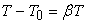the cooling water temperature varying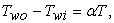it yields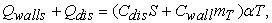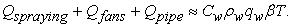Then taken Laplace transformation to (9) and combining (10), it yields the transfer function between the tunnel temperature T(s) and the MCT modules’ control signal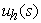as follows: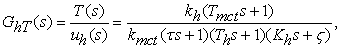(11)

where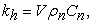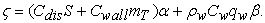### 4. System Controllers Design

According to the section III models, in this section, different control strategies will be present for systems control.

Combining the testing experience with the influence of high speed nitrogen gas flow to control system, a gas flow speed factor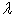is introduced to temperature control of the HSNWT, which the factor is selected as follows: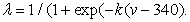(12)

v is gas flow speed and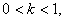a testing factor is shown by Figure 3.

Considering the HSNWT temperature control modeling nonlinear, uncertainties and disturbance, combined the gas flow speed factor, this paper proposed expert predictive and fuzzy PID strategies to achieve high variable temperature control, the closed-loop overall system control scheme is designed as the block diagram shown in Figure 4.

• Figure 3. The diagram of gas flow speed factor

The linguistic variable sets are established for the inputs and outputs of the fuzzy PID, which are chosen as {NB, NM, NS, ZO, PS, PM, PB} and expressed by e, ec={-5,-4,-3,-2,-1, 0, 1, 2, 3, 4, 5}. The incremental PID gains are determined with a defuzzier, the member-ship function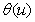for the fuzzy control output of each fuzzy rule can be determined as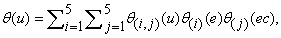(13)

where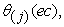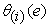and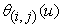denote the normal distribution membership functions. With wind speed factor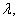the output of the fuzzy PID controller after defuzzier is calculated as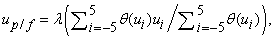(14)

that is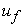and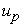control signals.

Considering the inner part of HSNWT temperature modeling uncertainties and disturbance, based on the testing tunnel temperature simplified model (11), an expert predictive PID control strategy is established to overcome the influences of the temperature inertial and transport time delay in the outer-loop. By selecting PID parameters according the varying trend of the temperature T(t) difference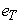and deviation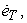furthermore, using the error of the actual temperature and the predicted temperature to compute control commands, the controller structure is shown in Figure 5.

In Figure 5 the k-th error sampled-data filtering output is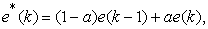(15)

where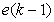is the (k-1)-th filtering output,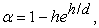h is sample time, d is constant time. There are five cases for the expert decision processing of the temperature.

Case 1: if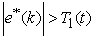, then the controller output is max (or min), the control system is equivalent to opened-loop control;

Case 2: if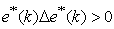and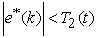, the error is varying to absolute value increase, then the controller is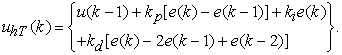(16)
• Figure 4. The closed-loop system control scheme
• Figure 5. The expert predictive PID controller structure

Case 3: if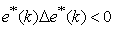and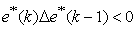or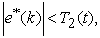the error is varying to absolute value decrease or reaches equilibrium state, then the controller output is similar to the (16);

Case 4: ifand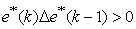andthen the controller output is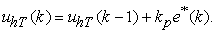(17)

Case 5: if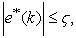the error absolute value is to be enough small, then the controller adds integration part, reduces the stable error.

### 5. Control Effective Analysis

In this section, in order to evaluate the designed system’s effectiveness, simulations and applications were performed in the HSNWT, respectively.

5.1. Simulation and Comparative Numerical Studies

For simulating, the temperature step responses is initially set to 800°C, then after 40 seconds bumped up to 1200°C,the relevant parameters of plant and testing tunnel see the Table I, the sampling period is 0.1s. The designed control law is evaluated numerically in the following cases.

1) We first conduct the set point and selecting the gas flow speed factor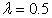for simulation, the system simulating results which compared with classical control methods are shown in Figure 6. In this paper, applying expert predictive PID control the temperature gets the set point within 10 seconds.

2) The temperature and water flow measuring instruments exist time delays, assuming the delay is 3 seconds, Figure 7 shows the control adjusting time becomes more than Figure 6, the overshoot and oscillation deteriorated of traditional PID techniques control temperature get the set points.

3) Without the gas flow speed factor, set the same point regulation control for an HSNWT testing simulation seen the Figure 8. The control performances compared with Figure 6 shows that the system overshoot and oscillation increase, meanwhile, the adjusting time becomes larger to achieve the set points than that of the control results with gas flow speed factor.

4) Analysis of model parameters perturbation, assuming the perturbation of model parameters are positive and negative 5% respectively, in Figure 9. The simulating results show that the temperature control needs differently time to reach steady state and effectively to cope with system modeling perturbation.

5) It is necessary for the HSNWT testing with different wind speed, in this case, the simulating gas flow speed which set three values, the Figure 10 shows that the gas wind speed increases much more the temperature control adjusting time becomes less, due to the high speed wind generate more heat energy in the HSNWT, which demonstrates that it is necessary to introduce a gas flow speed factor for system effectively control.

• Figure 6. Comparison of the traditional and fuzzy control
• Figure 7. Effect of time delay is 3 seconds
• Figure 8. Without gas flow speed factor effect
• Figure 9. Effect of modeling parameters perturbation
• Figure 10. Differently gas flow speed
• Figure 11. Gas flow speed variable at the time 80s

6) Disturbance rejection simulating, a disturbance, which is set to 0.4 V for the input signal of M430, is added to the inner-loop system at the 80 seconds, in Figure 11 the simulation results show the designed control strategy is better than that of classical control methods for attenuating the disturbance.

5.2. An application testing

In the specific process of an 0.3m radius HSNWT, there is a need for the temperature to be maintained between 800°C and 1200°C, a generic diagram of the process that applies to all components of the HSNWT in Figure 1.

In the testing, the heat exchanging system control process of all the electric actuators can be operated with manual and automatic control modes, conveniently, safety and reliable for user operating experiment. A steady state experiment is executed, which the control parameters are shown in HMI (see the Figure 12), the variable trends of temperature and cooling water pressure are monitored in HMI as the Figure 13 shown. The designed controllers are robustly and effectively for the HSNWT.

• Figure 12. Main operation interface
• Figure 13. Trends of temperature and water pressure

### 6. Conclusion

In this study, a cascade temperature control is proposed and validated on an high speed nitrogen gas wind tunnel testing (HSNWT). The proposed strategy chooses Siemens PLC and functional modules to build the control hardware system, designed expert predictive PID control the nitrogen gas heaters power and double fuzzy PID control cooling water flow and temperature, the control methods overcome the temperature modeling uncertainties and disturbance of HSNWT. Closed-loop performance is tested through simulation and experiment to show its effectiveness, when the temperature changes with different loading heat modes, and has excellent capability of variable high speed nitrogen gas flow.

### Acknowledgements

This work was supported by the Jiangsu province overseas research & training program for university prominent young & middle aged teachers & president, also supported in part by a grant from the National Nature Science Foundation No. 61174066.

### References

  Jewel B., Low speed wind tunnel testing, New York, Wiley, 1999. In article  S. Zhao, L. Liao, and Y. Chen, “1700°C Hot wind tunnel for thermal calibration,” Aviation Measure Testing Technology, 20(4). 3-6, 2000. In article  T. Xu, X. Pu, and Z. Yuan, “Application of PID parameter setting based on a genetic algorithm in a high-temperature multiphase flow wind tunnel,” Journal Engineering Thermal Energy Power, 25(4). 414-417, 2010. In article  Y. H. Li, Ch. Zh. Cai, Kok-M. Lee and F. J. Teng, “A Novel Cascade Temperature Control System for a High-Speed Heat-Airflow Wind Tunnel,” IEEE/ASME Transaction on Mechatronics, 18(4). 1310-1319, 2013. In article View Article  M. Salah, T. Mitchell, J. Wagner, and D. Dawson, “A smart multiple-loop automotive cooling system-Model, control, and experimental study,” IEEE/ASME Transactions on Mechatronics, 15(1). 117-125, 2010. In article View Article  Y. Li, S. S. Choi, and C. Yang, “Dish-Stirling Solar Power Plants: Modeling, Analysis, and Control of Receiver Temperature,” IEEE Transaction on Sustainable Energy, 5(2). 398-408, 2014. In article View Article  X. F. Li, D. H. Li, J. M. Gao, M. Pang, “Temperature drift compensation algorithm based on BP and GA in quartz flexible accelerometer,” Applied Mechanics and Mechanical Engineering, 249(25). 95-99, 2013. In article  R. D. Zhang, A. K. Xue, and F. R. Gao, “Temperature Control of Industrial Coke Furnace Using Novel State Space Model Predictive Control,” IEEE Transaction on Industrial Informatics, 10(4). 2084-2093, 2014. In article View Article  Emadi, A. Saboonchi, M. Taheri, and S. Hassanpour, “Heating characteristics of billet in a walking hearth type reheating furnace,” Applied Thermal Engineering, 63(1). 396-405, 2014. In article View Article  L. Han and Z. Zhang, “The application of immune genetic algorithm in main steam temperature of PID control of BP network,” Physics Procedia, 24. 80-86, 2012. In article View Article  J. H. Qiao, T. Y. Chai, “Intelligence-Based Temperature Switching Control for Cement Raw Meal Calcination Process,” IEEE Transactions on Control Systems Technology, 23(2). 644-661. 2015. In article View Article  L. Shen, J. J. He, Ch. H. Yang, etc.., “Temperature Uniformity Control of Large-Scale Vertical Quench Furnaces for Aluminum Alloy Thermal Treatment,” IEEE Transaction on Control Systems Technology, 24(1). 24-40, 2016. In article View Article

Published with license by Science and Education Publishing, Copyright © 2018 Yongbo Lai, Dewen Kong and Zhiwei WangThis work is licensed under a Creative Commons Attribution 4.0 International License. To view a copy of this license, visit http://creativecommons.org/licenses/by/4.0/